## Electrochemistry

1. Chemical symbols; ion charges of the most common ions
2. Electrochemical series
3. Oxidation and reduction
4. Redox reactions
5. Electrolysis
6. Electrode reactions and the PANC rule
7. Cation and anion
8. Electrolysis of various salt solutions. What happens at the cathode and anode?
9. How is nickel plated or zinc plated?
10. Galvanic Elements (Batteries)
11. Effects explained by the electrochemical series
12. Questions

Chemical symbols of the most common atoms; ion charges of the most common ions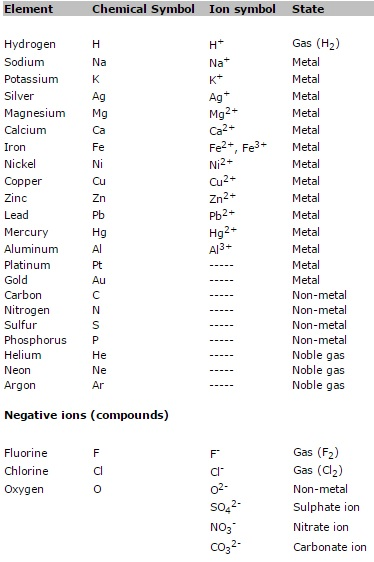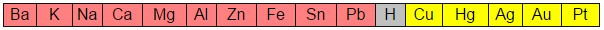Electrochemical series:

All metals in the periodic table are actually included in the electrochemical series. In the electrochemical series above are the metals that we are to work with. On the far left are the base metals and the farther right you go, the nobler the metals become. The metals to the left of hydrogen (H) are called base metals, while the metals to the right are called noble metals. If two different metals compete for electrons, the noblest will win. All acids contain hydrogen ions (H+) that dissolve base metals into ions. Noble metals are not affected by acid attack.

Oxidation and reduction

Oxidation means that an atom or ion gives away one or more electrons, thereby increasing the electrical charge, e.g. oxidation of iron into iron ions.Reduction means that an atom or ion adds one or more electrons thus reducing the electrical charge, e.g. reduction of copper ions into copper.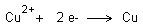Redox reactions

When an oxidation takes place there is always a simultaneous reduction. This is the basis of redox reactions. If we look at the examples above, an oxidation can only occur when a reduction occurs. If iron is immersed in copper ions (copper sulphate) then both reactions occur simultaneously. The reactions can be written together as follows.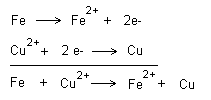If you want to show electron transfer, this is done as follows.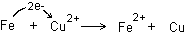Copper is nobler than iron and copper therefore wins the fight for the electrons

Electrolysis

With the help of electricity one can drive reactions. Below is the electrolysis of hydrochloric acid (HCl). At the negative terminal (cathode) hydrogen is created and at the positive terminal (anode) chlorine gas is created. The hydrogen ignites by the heat of a match (about 500 °C) and the chlorine gas formed is toxic. The electrodes consist of graphite.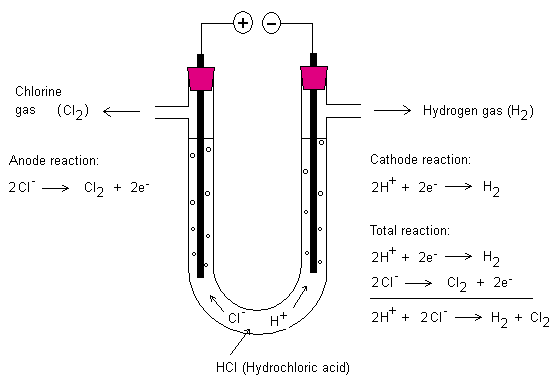Electrode Reactions and the PANC-rule

Above are electrode reactions for the electrolysis of hydrochloric acid. The positive terminal is an electrode and the negative terminal is also an electrode. At these electrodes chemical reactions occur. If the electrode is the positive terminal it is called anode. The negative terminal is called cathode.

To help you remember which electrode is which one can make use of the PANC-rule. PANC stands for positive anode negative cathode. The cathode is negative because the battery above has electrons in the surplus that can be delivered here.

The anode is positive since there is a deficit of electrons here. Negative ions want to go to the anode. In the above case the chloride ions (Cl-) want to go to the anode to deliver one electron each. Chlorine gas is formed. Each chlorine atom pairs up with another chlorine atom. This allows the two chlorine atoms feel that they have eight electrons each in their respective outermost shell. A covalent bond is created and the molecule created is called the chlorine molecule (Cl2).

Likewise at the cathode two hydrogen atoms pair up together to form a hydrogen molecule (H2). This happens when each hydrogen ion is added one electron.

Cation and anion

The ions wanting to the cathode (negative terminal) are called cations, e.g. Na+, Mg+2, Ca+2, Cu+2, Zn+2, Al+3, i.e. all positive ions.

Ions wanting to the anode (positive terminal) are called anions, e.g. Cl-, F-, SO4-2, CO3-2, i.e. all negative ions.

Electrolysis of various salt solutions ... what happens? Know theoretically what happens when different solutions are electrolyzed. What will happen to the cathode and anode?

Below is electrolysis of copper chloride.How is nickel plated or zinc plated?

This can be done by means of electrolysis. Use zinc ions (Zn2+) or nickel ions (Ni2+) in solution. Let the metal object that is to be zinc plated or nickel plated act as negative terminal (cathode). Use the DC and start the process. Electrons will then be located here and reduce zinc or nickel ions to zinc or nickel.

Galvanic Elements (Batteries)

All the metals in the electrochemical series have different abilities to oxidize other metals, i.e. get the other metal to give away electrons that they want themselves. A metal that oxidizes another metal is known as an oxidizing agent and the metal that loses the electron or electrons is then oxidized.

Similarly, a metal can be a reducing agent if it instead gives away electrons to another metal. The other metal is reduced, i.e. has got a lower charge.

E.g.Copper ions: oxidizing agent (oxidizes iron atoms). The copper ions are reduced to copper.
Iron: reducing agent (reduces copper ions). The iron atoms are themselves oxidized to iron ions.

You can let the two metals compete in the “boxing ring” and fight for the electrons. The noblest metal will win the electrons and the weaker will lose theirs. The different ability of the metals to add electrons is measured in comparison to hydrogen, which constitutes the border between noble and base metals. The capacity is measured in volts (voltage). The two metals are compared on the capacity and there will be an electron current in the conduit between the electrodes.

Both metal pieces in the liquid below are made of two different metals. The electrode giving electrons to the other metal trough the conduit is the negative terminal (cathode). The electrode receiving electrons is the positive terminal (anode). The voltmeter will show a voltage. We have created a battery. If one of the electrodes would have been copper (anode) and the other would have been iron (cathode), the voltmeter would have shown approximately 0.3 to 0.4 V. If one had been a silver electrode (anode) and the other a zinc electrode (cathode), the voltmeter would have shown approximately 1.50 to 1.60 V. The ion concentration between the electrodes also affects to some extent what voltage there will be between the electrodes. From table collections one can calculate the voltage between electrodes of different metal types. Here, the ion concentration in the electrolyte is often 1 mol/dm3 (1 molar) and the atmospheric pressure is 1 bar.Effects explained by the electrochemical series

If you are to nail a copper roof, you should use copper nails. If you use iron nails, then the iron will donate electrons to the copper as described above. There is always some moisture with salts between the copper and the nails of the roof. A galvanic current will be created, and iron nails will oxidize into iron ions.

Questions:

1. Write the chemical symbol of the sulphate ion, nitrate ion and carbonate ion?
2. What does the electrochemical series show?
3. Why can we arrange all the metals in the electrochemical series - what capacity is compared?
4. What metals are base?
5. What metals are noble?
6. What is the border between noble and base metals?
7. What is oxidation?
8. What is reduction?
9. What is a redox reaction - give also an example of a redox reaction. Show also the electron transfer.
10. Describe the electrolysis of hydrochloric acid? Draw the layout and write down the electrode reactions and the total reaction.
11. How could you test what gases are formed at the two terminals in the question above?
12. Describe the electrolysis of copper chloride? Draw the layout and write down the electrode reactions and the total reaction.
13. How could you see what substances are formed at the two terminals in the question above?
14. What is a galvanic element?
15. What do both metals compete about in a galvanic battery?
16. How do you measure that?
17. Which electrode (anode or cathode) will always win electrons?
18. Which electrode (anode or cathode) will always lose electrons?
19. Why should you nail a copper roof with copper nails?
20. Why should you not nail an iron roof with copper nails?
21. How do you cover a metal-object with zinc?

• NGU Science
• JAN 1, 2012
• JAN 25, 2012